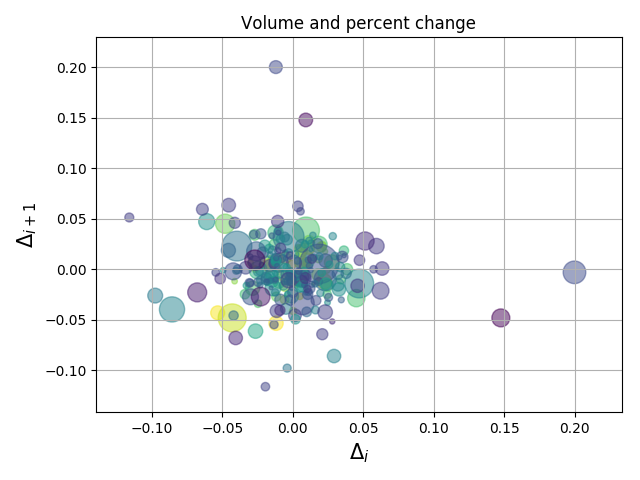You are reading an old version of the documentation (v2.2.3). For the latest version see https://matplotlib.org/stable/gallery/lines_bars_and_markers/scatter_demo2.html

# Scatter Demo2¶

Demo of scatter plot with varying marker colors and sizes.```import numpy as np
import matplotlib.pyplot as plt
import matplotlib.cbook as cbook

# Load a numpy record array from yahoo csv data with fields date, open, close,
# volume, adj_close from the mpl-data/example directory. The record array
# stores the date as an np.datetime64 with a day unit ('D') in the date column.
with cbook.get_sample_data('goog.npz') as datafile:
price_data = np.load(datafile)['price_data'].view(np.recarray)
price_data = price_data[-250:]  # get the most recent 250 trading days

delta1 = np.diff(price_data.adj_close) / price_data.adj_close[:-1]

# Marker size in units of points^2
volume = (15 * price_data.volume[:-2] / price_data.volume)**2
close = 0.003 * price_data.close[:-2] / 0.003 * price_data.open[:-2]

fig, ax = plt.subplots()
ax.scatter(delta1[:-1], delta1[1:], c=close, s=volume, alpha=0.5)

ax.set_xlabel(r'\$\Delta_i\$', fontsize=15)
ax.set_ylabel(r'\$\Delta_{i+1}\$', fontsize=15)
ax.set_title('Volume and percent change')

ax.grid(True)
fig.tight_layout()

plt.show()
```

Keywords: matplotlib code example, codex, python plot, pyplot Gallery generated by Sphinx-Gallery